Latest Banking jobs   »

# Quantitative Aptitude Quiz For SBI PO Prelims 2022- 13th December

Directions (1-5): Bar graph given below show total number of students in five different schools (A, B, C, D & E) in two different years (2021, 2022). Read the following bar graph carefully and answer the questions given below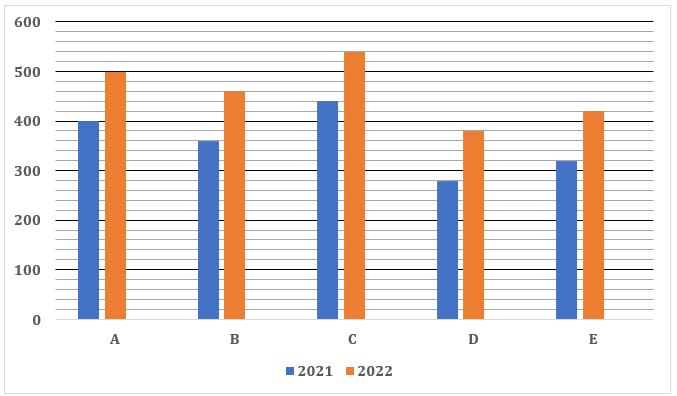Q1. The ratio of number of boys to girls in school B and that in D in 2021 is 11:7 & 9:5 respectively. Find the difference between total number of boys in B & D together and number of total girls in both schools in 2021?
(a) 120
(b) 160
(c) 180
(d) 240
(e) 200

Q2. Find the total number of students in school A in 2022 is what percent (approximately) more or less than total number of students in school C in 2021?
(a) 14%
(b) 18%
(c) 26%
(d) 22%
(e) 8%

Q3. If total number of students in school F in 2022 is 66.66% more than total number of students in E in 2022, then find total number of students in school D and F together in 2022?
(a) 1180
(b) 1140
(c) 1120
(d) 1080
(e) 1160

Q4. Find the ratio of total number of students in E and that in C together in 2022 to total number of students in B and that in D in 2021?
(a) 5:2
(b) 3:7
(c) 3:5
(d) 4:7
(e) 3:2

Q5. Average number of students in A, B & C in 2022 is how much more or less than average number of students in D & E in 2021?
(a) 240
(b) 200
(c) 160
(d) 180
(e) 280

Directions (6-10): Given below is the table which shows the percentage of boys in two school A and B in five different years. Read the data carefully and answer the questions.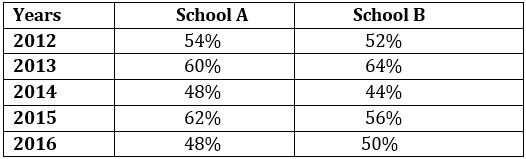Note: Total students in any school = Total boys + Total girls in each school

Q6. In 2012, ratio of boys in school A to school B is 45 : 52 and total students in both school in 2012 is 1100. Find the total number of girls in both school in same year.
(a) 568
(b) 528
(c) 518
(d) 418
(e) 488

Q7. If in 2014, boys in school A and B are 288 and 264 respectively then, find total number of girls in both school in 2014.
(a) 594
(b) 640
(c) 564
(d) 648
(e) 630

Q8. If boys in school A in 2014 and girls in school B in 2012 are equal then boys in school B in 2012 are what percent of girls in school A in 2014.
(a) 85%
(b) 95%
(c) 90%
(d) 80%
(e) 100%

Q9. In 2016, girls in school A are 16⅘% less than girls in school B. Find the ratio of boys in school A to that of school B in 2016.
(a) 100 : 123
(b) 98 : 117
(c) 98 : 125
(d) 92 : 117
(e) 96 : 125

Q10. If total students in school A in 2015 and total student in B in 2013 are 700 and 400 respectively, then find the average number of boys in school A in 2015 and boys in school B in 2013.
(a) 344
(b) 345
(c) 348
(d) 368
(e) 358

Directions (11-15): Given bar graph shows the data of expenses (in % distribution) of Mr. Chunky in 4 months on rent, travel & food. Study the graph carefully and answer the questions.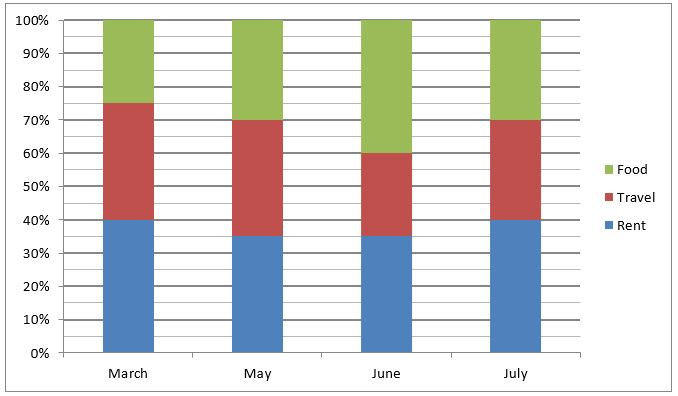Q11. If salary of Mr. Chunky is Rs. 12000 in July and his savings is half of his expenditure on rent. Find his expenditure on food. (in Rs. )
(a) 3500
(b) 2000
(c) 4000
(d) 3000
(e) 4500

Q12. If savings and salary of Mr. Chunky are same for all the given months then expenditure on travel in March is what percent of expenditure on food in June?
(a) 87.5%
(b) 85%
(c) 90%
(d) 92.5%
(e) None of these

Q13. If ratio of total expenditure in May & July is 5:4. Find ratio of expenditure on rent in May to expenditure on travel in July.
(a) 3:2
(b) 6:7
(c) 7:6
(d) 24:35
(e) 35:24

Q14. Income of Chunky in March & July is Rs. 5000 & Rs. 8000 of which he saves only 10% in each month. What is his average expenditure on rent in these 2 months?
(a) Rs.2400
(b) Rs.2300
(c) Rs. 2340
(d) Rs.2430
(e) Rs.2360

Q15. Expenditure on travel in May is what percent more than expenditure on travel in July if total expenditure for both the months is same?
(a) 15%
(b) 12.5%
(c) 16.67%
(d) 20%
(e) 10%

Solutions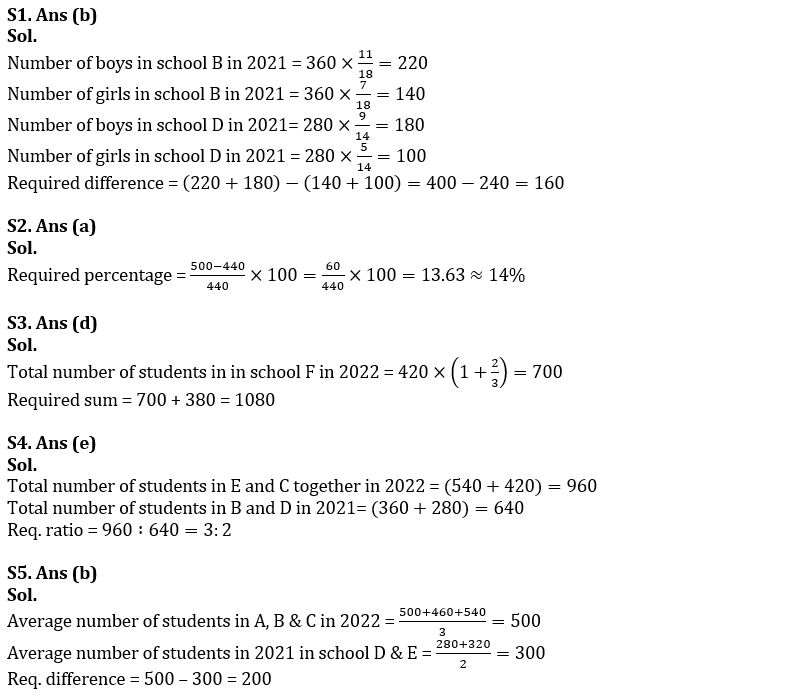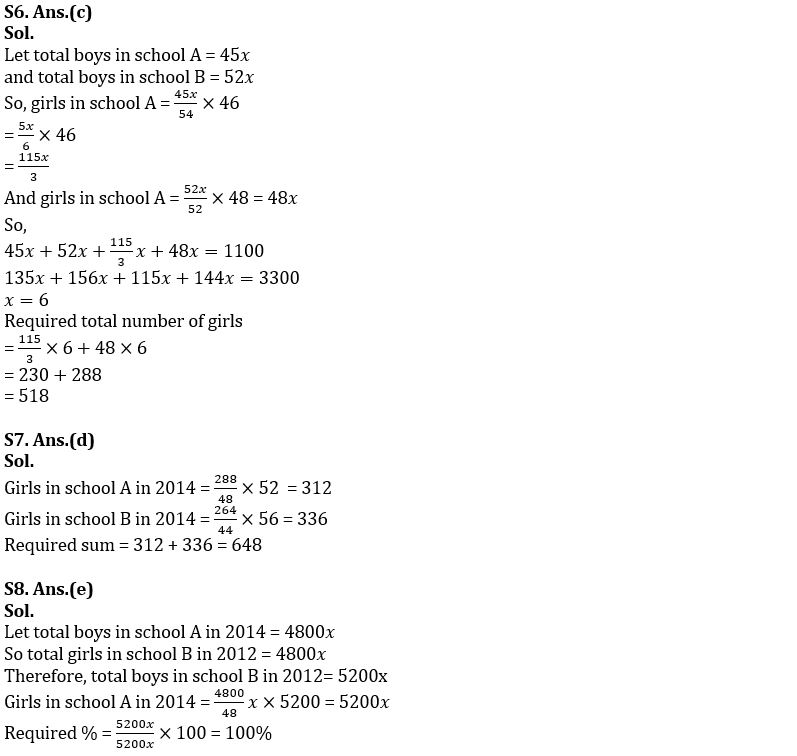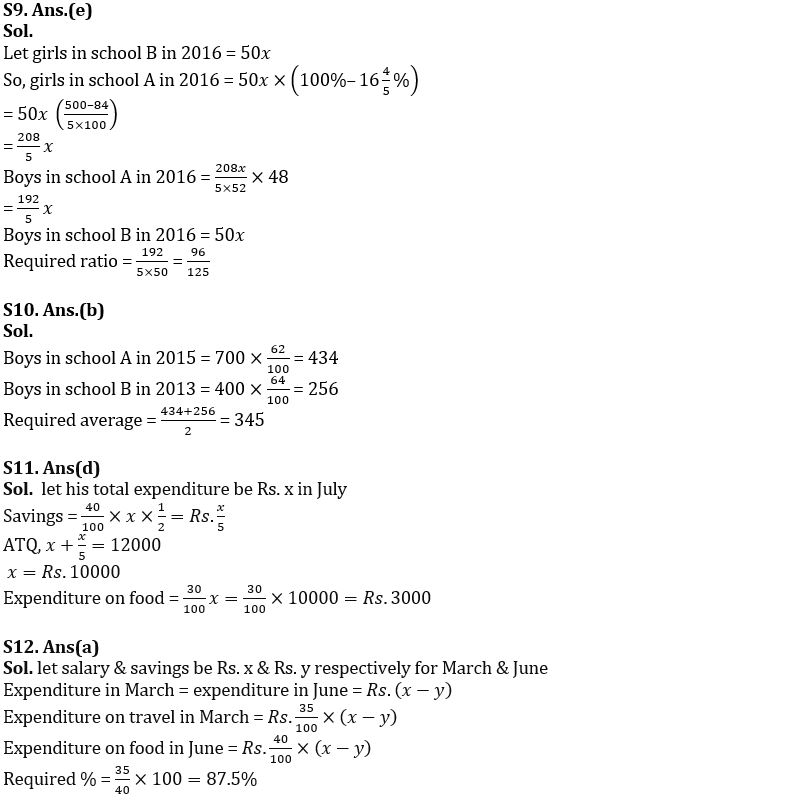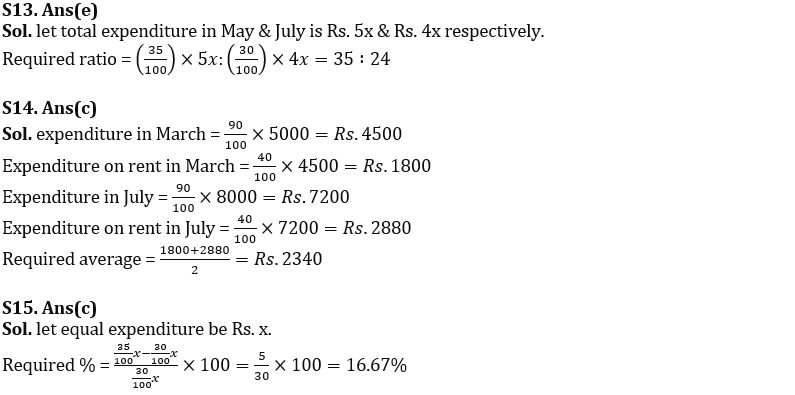#### Congratulations!Union Budget 2023-24: Free PDF